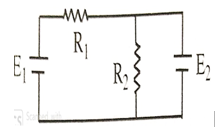Two cells of emf E1  and E2 are connected to two resistors  R1 and R2  as shown. If E2   is short circuited then currents through   R1 and R2  are

# Two cells of emf E1  and E2 are connected to two resistors  R1 and R2  as shown. If E2   is short circuited then currents through   R1 and R2  are1. A

$\frac{{\mathrm{E}}_{1}}{{\mathrm{R}}_{2}},0$

2. B

$0,\frac{{\mathrm{E}}_{1}}{{\mathrm{R}}_{2}}$

3. C

$\frac{{E}_{1}}{{R}_{1}},0$

4. D

$0,\frac{{\mathrm{E}}_{1}}{{\mathrm{R}}_{1}}$

Register to Get Free Mock Test and Study Material

+91

Verify OTP Code (required)

### Solution:

After short circuiting, R2 becomes meaningless. Current will not flow through R2

So, current will be $\frac{{\mathrm{E}}_{1}}{{\mathrm{R}}_{1}}$Register to Get Free Mock Test and Study Material

+91

Verify OTP Code (required)